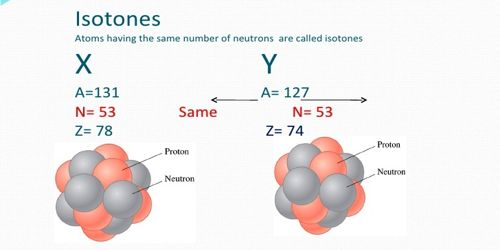Physics

# IsotonesIsotones: Those atoms which contain a same number of neutrons (N), but a different number of protons (Z), they are called isotones. They are the atom of a different element which contains the same number of a neutron with the different mass number and atomic number. Two nuclides are called isotones if they have the same neutron number N, but different proton numbers Z. For example, Boron- 12 and Carbon-13 nuclei, but both contain 7 neutrons. The atomic number and the mass number are different. Most atoms having an odd number of neutrons are isotonic in nature which in turn establishes the fact that odd neutron configurations are relatively more stable than versa.

For example, 40Ca20 and 39K19 i.e., calcium and potassium both have the same number of neutrons, they are 20. Hence they are isotones.Another Example, silicon, and phosphorous have the same number of neutron 16 with different mass number 30, 31 and atomic number 14, 15. The numbers of the naturally occurring isotones provide an insight into the stability of a particular neutron configuration.